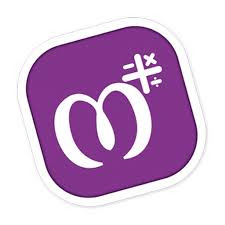## Download MAthry A way of Learning Math Supper Application from here

Speed up mathematical calculations with Mathry!

A Simple yet amazing math application for students, teachers and parents.

Increase your brainpower using thousands of mathematical questions/quiz.Excellent for preschool and grade school kids to learn basic arithmetic ideas like Addition, Subtraction, Multiplication, Division, Decimals, Fractions, pure mathematics so on.

Meant for students, this app helps students to pick up all mathematic operations- quite easily. You will find several interesting exercises and games to give kids ample practice.

Teachers love the app because it is easily accessible anytime, anywhere; and include worksheet and quizzes too.Learn these basic operations:
✓ Subtraction
✓ Multiplication
✓ Division

Other Features:
☆ Worksheet Generator (Teachers or Parents can use for an exam)
☆ Basic Operations on Number Base
☆ Fractions and Decimals
☆ Mixed Operators
☆ Percentage
☆ Square
☆ Square Root
☆ Cube
☆ Cube Root
☆ Find Missing
☆ and Much More!

Languages available:
» English
» Spanish
» Hindi
» Gujarati